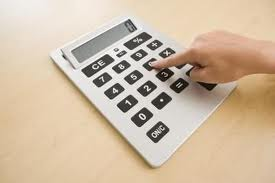Introduction:

Central tendency is a single value that attempts to describe a set of data by identifying the central position within that set of data. As such, measures of central tendency are sometimes called measures of central location. They are also classed as summary statistics. The mean (often called the average) is most likely the measure of central tendency that you are most familiar with, but there are others, such as the median and the mode.

The mean, median and mode are all valid measures of central tendency, but under different conditions, some measures of central tendency become more appropriate to use than others. In the following sections, we will look at the mean, mode and median, and learn how to calculate them and under what conditions they are most appropriate to be used.

Mean

The mean is the best-known and most widely used measure of central tendency. It is what most people call the “average.” It is used to describe the distribution of interval-ratio variables such as age, income, and education.

The mean is calculated by adding up all the scores and dividing the result by the number of scores in the distribution. For example, if five families have 0, 2, 2, 3, and 5 children respectively, the mean number of children is (0 + 2 + 2 + 3 + 5)/5 = 12/5 = 2.4. This means that the five households each have an average of 2.4 children.

Median

The median represents the exact middle of a distribution so that half of the cases are above it and half are below it. It is the middle case in a distribution when the scores are arranged in order from lowest to highest.

For example, let’s suppose we have the following list of numbers: 5, 7, 10, 43, 2, 69, 31, 6, 22. First, we must arrange the numbers in order from lowest to highest. The result is this: 2, 5, 6, 7, 10, 22, 31, 43, 69. The median is 10 because it is the exact middle number. There are four numbers below 10 and four numbers above 10.

MODE

Mode is defined as the value that occurs most frequently in the data. Some data sets do not have a mode because each value occurs only once. On the other hand, some data sets can have more than one mode. This happens when the data set has two or more values of equal frequency which is greater than that of any other value. Mode is rarely used as a summary statistic except to describe a bimodal distribution. In a bimodal distribution, the taller peak is called the major mode and the shorter one is the minor mode.